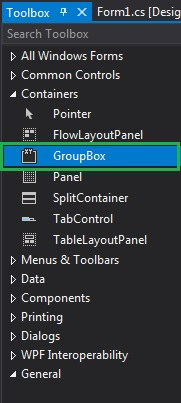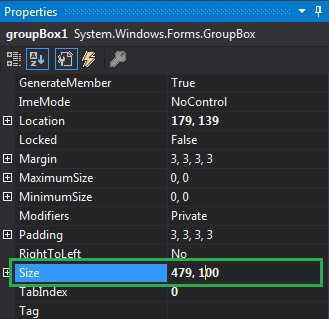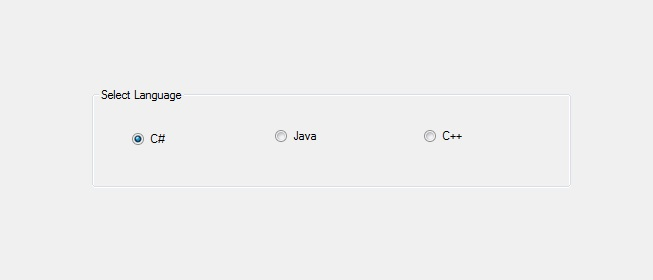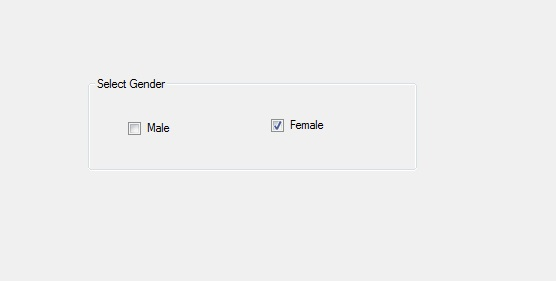Skip to content
Related Articles
How to set the Size of the GroupBox in C#?
• Last Updated : 29 Jul, 2019

In Windows form, GroupBox is a container which contains multiple controls in it and the controls are related to each other. Or in other words, GroupBox is a frame display around a group of controls with a suitable optional title. Or a GroupBox is used to categorize the related controls in a group. In GroupBox, you can set the size of the GroupBox in the form using the Size Property.
This property represents both the height and width of the GroupBox in pixels. You can set this property in two different ways:

1. Design-Time: It is the easiest way to set the size of the GroupBox as shown in the following steps:

• Step 1: Create a windows form as shown in the below image:

Visual Studio -> File -> New -> Project -> WindowsFormApp• Step 2: Next, drag and drop the GroupBox from the toolbox to the form as shown in the below image:• Step 3: After drag and drop you will go to the properties of the GroupBox and set the size of the GroupBox as shown in the below image:Output:2. Run-Time: It is a little bit trickier than the above method. In this method, you can set the size of the GroupBox programmatically with the help of given syntax:

`public System.Drawing.Size Size { get; set; }`

Here, Size indicates the height and width of the GroupBox in pixels. The following steps show how to set the size of the GroupBox dynamically:

• Step 1: Create a GroupBox using the GroupBox() constructor is provided by the GroupBox class.
```// Creating a GroupBox
GroupBox gbox = new GroupBox();
```
• Step 2: After creating GroupBox, set the Size property of the GroupBox provided by the GroupBox class.
```// Setting the size
gbox.Size = new Size(329, 94);
```
• Step 3: And last add this GroupBox control to the form and also add other controls on the GroupBox using the following statements:
```// Adding groupbox in the form
this.Controls.Add(gbox);

and

// Adding this control
// to the GroupBox
gbox.Controls.Add(c2);
```

Example:

 `using` `System;``using` `System.Collections.Generic;``using` `System.ComponentModel;``using` `System.Data;``using` `System.Drawing;``using` `System.Linq;``using` `System.Text;``using` `System.Threading.Tasks;``using` `System.Windows.Forms;`` ` `namespace` `WindowsFormsApp45 {`` ` `public` `partial` `class` `Form1 : Form {`` ` `    ``public` `Form1()``    ``{``        ``InitializeComponent();``    ``}`` ` `    ``private` `void` `Form1_Load(``object` `sender, EventArgs e)``    ``{``        ``// Creating and setting properties``        ``// of the GroupBox``        ``GroupBox gbox = ``new` `GroupBox();``        ``gbox.Location = ``new` `Point(179, 145);``        ``gbox.Size = ``new` `Size(329, 94);``        ``gbox.Text = ``"Select Gender"``;``        ``gbox.Name = ``"Mybox"``;`` ` `        ``// Adding groupbox in the form``        ``this``.Controls.Add(gbox);`` ` `        ``// Creating and setting ``        ``// properties of the CheckBox``        ``CheckBox c1 = ``new` `CheckBox();``        ``c1.Location = ``new` `Point(40, 42);``        ``c1.Size = ``new` `Size(49, 20);``        ``c1.Text = ``"Male"``;`` ` `        ``// Adding this control``        ``// to the GroupBox``        ``gbox.Controls.Add(c1);`` ` `        ``// Creating and setting ``        ``// properties of the CheckBox``        ``CheckBox c2 = ``new` `CheckBox();``        ``c2.Location = ``new` `Point(183, 39);``        ``c2.Size = ``new` `Size(69, 20);``        ``c2.Text = ``"Female"``;`` ` `        ``// Adding this control ``        ``// to the GroupBox``        ``gbox.Controls.Add(c2);``    ``}``}``}`

Output:My Personal Notes arrow_drop_up